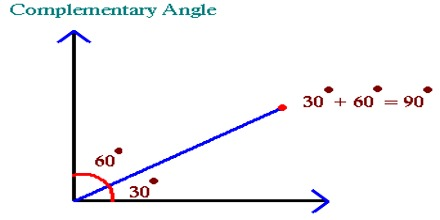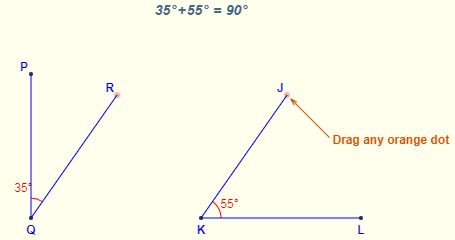Complementary Angles

Two angles are complementary if the sum of their angles equals 900. A common case is when they form a right angle.

If one angle is known, its complementary angle can be found by subtracting the measure of its angle from 900. Complementary angles form a right angle (L shape) and have a sum of 90 degrees.Example: What is the complementary angle of 430?

Solution: 900 – 430 = 470In the figure above, the two angles ∠PQR and ∠JKL are complementary because they always add to 90° Often the two angles are adjacent, in which case they form a right angle.

In a right triangle, the two smaller angles are always complementary. (Why? – one angle is 90° and all three add up to 180°. Therefore the two smaller ones must add to 90° and so are complementary by definition).